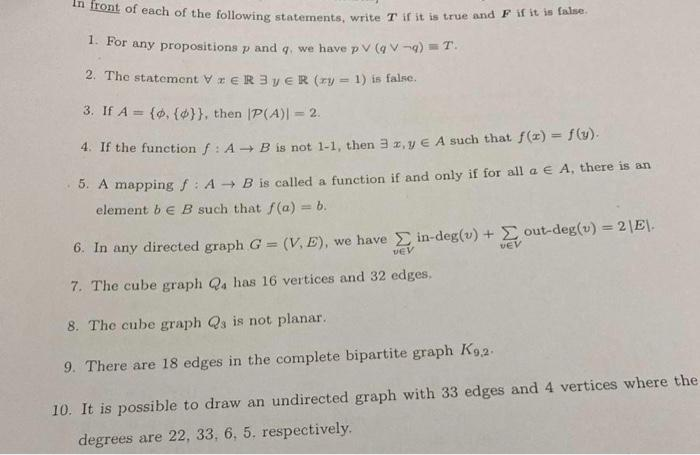# (Solved): ans only 1. For any propositions $$p$$ and $$q$$, we have $$p \vee(q \vee \neg q)=T$$. 2. The ...

ans only1. For any propositions $$p$$ and $$q$$, we have $$p \vee(q \vee \neg q)=T$$. 2. The statement $$\forall x \in \mathbb{R} \exists y \in \mathbb{R}(x y=1)$$ is false. 3. If $$A=\{\phi,\{\phi\}\}$$, then $$|\mathcal{P}(A)|=2$$. 4. If the function $$f: A \rightarrow B$$ is not $$1-1$$, then $$\exists x, y \in A$$ such that $$f(x)=f(y)$$. 5. A mapping $$f: A \rightarrow B$$ is called a function if and only if for all $$a \in A$$, there is an element $$b \in B$$ such that $$f(a)=b$$. 6. In any directed graph $$G=(V, E)$$, we have $$\sum_{v \in V}$$ in-deg $$(v)+\sum_{v \in V} \operatorname{out-deg}(v)=2|E|$$. 7. The cube graph $$Q_{4}$$ has 16 vertices and 32 edges. 8. The cube graph $$Q_{3}$$ is not planar. 9. There are 18 edges in the complete bipartite graph $$K_{9,2}$$. 10. It is possible to draw an undirected graph with 33 edges and 4 vertices where the degrees are $$22,33,6,5$$. respectively.

We have an Answer from Expert